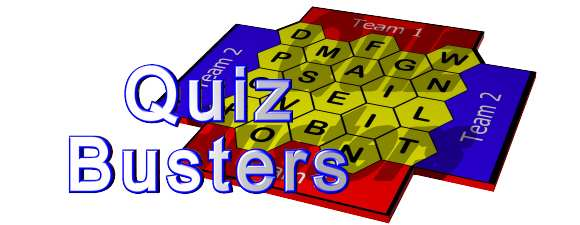# Quiz-Busters Games DirectorySearch for quizzes:
Subject:
Key Stage:

Found 15 results for Key Stage 2 Mathematics
Showing results 1 to 15

Using Brackets (Key Stage 2 - Mathematics)
Brackets involving addition, subtraction and multiplication.
Revision, spring 1 (Key Stage 2 - Mathematics)
Covers maths topics from spring 1, yr 3 of new strategy, eg. symmetry, shape and measure.
2D and 3D shapes (Key Stage 2 - Mathematics)
This quiz is about 2D and 3D shapes. It is aimed at year 3 pupils and can be used for a mental oral starter or plenary.
Length Weight Capacity (Key Stage 2 - Mathematics)
Revision of appropriate metric units to use when measuring length, weight and capacity.
Times Tables (x2 x3 x4 x5 x10) (Key Stage 2 - Mathematics)
Time Tables questions relating to the x2 x3 x4 x5 and x10 tables.
What number am i? (Key Stage 2 - Mathematics)
Numbers in words
Block B - Unit 1 - Year 6 - quiz 1 (Key Stage 2 - Mathematics)
Block B - Unit 1 - Year 6 - Shape and Space to help learn maths vocabulary.
A1 - year 6 - quiz 1 (Key Stage 2 - Mathematics)
Quiz to help learn the new maths vocabulary for block A - unit 1 of year 6.
Basic sums (Key Stage 2 - Mathematics)
Quiz using basic addition, subtraction and multiplication to practice mental arithmetic.
times tables (Key Stage 2 - Mathematics)
Practice your times tables up to 10 x 10
Year 3 Division Quest (Key Stage 2 - Mathematics)
Division with no remainders
Maths quiz on 3 and 4 times tables (Key Stage 2 - Mathematics)
3 and 4 times table facts.
Numeracy blockbusters (Key Stage 2 - Mathematics)
Shape quiz
SHAPE AND SPACE - Year 6 (Key Stage 2 - Mathematics)
Shape and space - involving key words and phrases that should be known in Year 6.
SHAPE AND SPACE - Year 6 (Key Stage 2 - Mathematics)
Shape and space - involving key words and phrases that should be known in Year 6.

Showing results 1 to 15